# Connecting the 3D DGS Calques3D with the CAS Maple

E. Roanes-Lozano , N. Van Labeke , E. Roanes-Macías

## Abstract

Many (2D) Dynamic Geometry Systems (DGSs) are able to export numeric coordinates and equations with numeric coefficients to Computer Algebra Systems (CASs). Moreover, different approaches and systems that link (2D) DGSs with CASs, so that symbolic coordinates and equations with symbolic coefficients can be exported from the DGS to the CAS, already exist. Although the 3D DGS Calques3D can export numeric coordinates and equations with numeric coefficients to Maple and Mathematica, it cannot export symbolic coordinates and equations with symbolic coefficients. A connection between the 3D DGS Calques3D and the CAS Maple, that can handle symbolic coordinates and equations with symbolic coefficients, is presented here. Its main interest is to provide a convenient time-saving way to explore problems and directly obtain both algebraic and numeric data when dealing with a 3D extension of "ruler and compass geometry". This link has not only educational purposes but mathematical ones, like mechanical theorem proving in geometry, geometric discovery (hypotheses completion), geometric loci finding... As far as we know, there is no comparable "symbolic" link in the 3D case, except the prototype 3D-LD (restricted to determining algebraic surfaces as geometric loci).

## 1 Introduction: CASs and DGSs

The first Computer Algebra Systems (CASs), Macsyma and Reduce, were designed in the sixties to cope with the symbolic problems of Astronomy and High Energy Physics. CASs are distinguished for using exact arithmetic (instead of the built-in floating point arithmetic) and for handling non-assigned variables (i.e., variables in the mathematical sense, not in the usual sense in Computer Science). Many extensions like symbolic differentiation and integration, linear and non-linear equation and polynomial system solving, 2D and 3D plotting... are usually included too.

Let us detail what “handling non-assigned variables” means.

Example 1 Any computer language can compute $(x + y)2 + (x − y)^2$ for any given values of $x$ and $y$. But when we ask our students to try to obtain a simpler expression for that polynomial, we are expecting them to perform symbolic computations involving “x” and “y”, without assigning values to these variables.

After expanding and cancelling, $4 · x · y$ should be reached. CASs can also handle such operations, whereas the typical computer languages cannot.

Example 2 An almost standard programming exercise is to write a program that, given $f(x)$ and $n \in \mathbb{Z} (n > 0)$, approximates $\int^a_b f(x) \ dx$ by dividing interval $(a,b)$ in $n$ intervals (there are different well-known methods). Meanwhile, a CASs can find (for most functions) $\int f(x) \ dx$, i.e., the antiderivative of $f(x)$.

This way CASs can treat problems of a higher degree of abstraction than usual computer languages.

The best known CASs are probably Mathematica [45,50], Maple [12,16,25 ,37,51], Derive [20 ,22,30] (recently discontinued) , Reduce [24 ,26,53], Axiom [17 ,54], MuPad  and Maxima (a descendant of the discontinued Macsyma) .

There are some specific purpose ones, like CoCoA  and Singular .

Dynamic Geometry Systems (DGSs) are interactive systems that allow to draw “ruler and compass” geometric constructions using only the mouse, and to deform the construction by dragging and dropping the points that do not depend on previously defined objects. The best-known 2D DGSs are probably Cabri Geometry , The Geometer’s Sketchpad , Cinderella [19 ,61] and GeoGebra [14,62], although many other exist. They are great tools for exploring classic geometry.

Both Computer Algebra Systems (CASs) and 2D DGSs have reached a high level of development. And even some 3D DGSs like Cabri3D and the free Calques3D , have recently been developed .

However, a gap between these two types of system still exists. On one hand, powerful packages devoted to Euclidean geometry have been implemented in CASs like Maple or Derive [20 ,37]. But they have incorporated neither mouse drawing capabilities, nor dynamic capabilities . On the other hand, well-known 2D DGSs (such as Cabri Geometry, The Geometer’s Sketchpad, Cinderella, GeoGebra, . . . ) do not provide algebraic facilities, i.e., they can handle numeric coordinates and equations with numeric coefficients but they cannot handle symbolic coordinates and equations with symbolic coefficients, something that we can summarize by saying that “they can’t handle parameters”.

Let us try to illustrate the difference with some examples.

Example 3 If point P lies by definition on line $y = x$, any DGS can return its coordinates at a certain instant, e.g. $(3,3)$ or $(8,8)$, but the well-known DGSs cannot return its general coordinates (just reflecting the membership to the line), i.e., something like $(k, k)$ ($k$ is the “parameter” in this case).

Example 4 If point P is a free point, any DGS can return the equation of the line through point $(0, 0)$ and P at a certain instant, e.g. $y = 2·x$ or $y = −3·x$, but the well-known DGSs cannot return the general equation of this “pencil of lines through point $(0, 0)$”, i.e., something like $y = a · x$ ($a$ is the “parameter” in this case).

Example 5 If points $P = (p1, p2)$, $Q = (q1, q2)$ and $R = (r1, r2)$ are free points, any DGS can return the (approximate) coordinates of the baricenter of triangle $PQR$ at any time, e.g. $(3.45730, 5.56112)$, but the well-known DGSs cannot return the coordinates of the baricenter of a general triangle as a function of the coordinates of its vertices, i.e. $$(\frac{p1+q1+r1}{3},\frac{p2+q2+r2}{3})$$ with the notation of this particular example ($p1$, $q1$, $r1$, $p2$, $q2$, $r2$ are the “parameters” in this case).

The fact that DGS should be able to handle parameters was underlined years ago by Tomás Recio , on the ground that it would allow DGS to treat problems of a higher degree of abstraction.

The aim of this paper is to describe one such attempt to bridge the gap between DGS and CAS in the context of 3D geometry. In Section 2, we briefly review how similar works have been done for 2D geometry. In Section 3, we describe preliminary works to support mechanical theorem proving in 3D geometry.

Finally, Section 4 demonstrates the potentials of such an approach by describing several applications of a connection between 3D DGS and CAS.

## 2 State-of-the-art in 2D DGSs - CASs Connection

In order to solve this lack of parameter handling in most DGSs and taking advantage of the symbolic possibilities of CASs, different solutions for connecting both environments have been implemented The possible strategies for collaboration followed by the different authors can be classified as follows [31,33]:

1. To develop a new system that integrates a new DGS and a new CAS. Examples:
• Geometry Expert [15,65],
• Java Geometry Expert [10,66],
• Geometry Expressions [43,67].
2. To develop a new DGS that can communicate with existing CASs. Examples:
• GEOTHER & Epsilon [44,68 ,69] → Maple,
• Geometry Expressions ↔ Maple, Mathematica
• GDI  → CoCoA, Mathematica.
3. To develop an external translator that allows an existing DGS to communicate with an existing CAS (this approach has the advantage of reusing software). Example:
• paramGeo , that connects The Geometer’s Sketchpad v.3, v.4 → Maple, Derive and includes the corresponding Derive and Maple geometric packages (developed “ad hoc”).

Note that Geometry Expressions appears both under i) and under ii) because it incorporates a small internal CAS but can also communicate with the external CASs Maple and Mathematica.

An impressive possibility of Geometry Expressions is to communicate bidirectionally with the external CASs (that is, the results of the CAS can make the construction in the DGS change!). That is the reason for typing a ↔ instead of a → in ii) above. It is, as far as we know, the only DGS with this surprising and powerful capability.

### 2.1 Some Examples of the 2D DGSs - CASs Connection

#### 2.1.1 Pythagoras Theorem at a Mouse Click with Geometry Expressions

We know that results related to Pythagoras theorem were known in ancient Egypt. For example, if a triangle has sides of length 3, 4 and 5, then it is a right angle triangle (what is very convenient, for example, for building corners). Babylonian mathematics, although had an impressive accuracy in fields like astronomy, also presented a lack of formalism.

All the well known DGSs (such as GeoGebra) can be used to find that the diagonal of a right angle triangle of sides 3 and 4 has length 5 (Figure 1).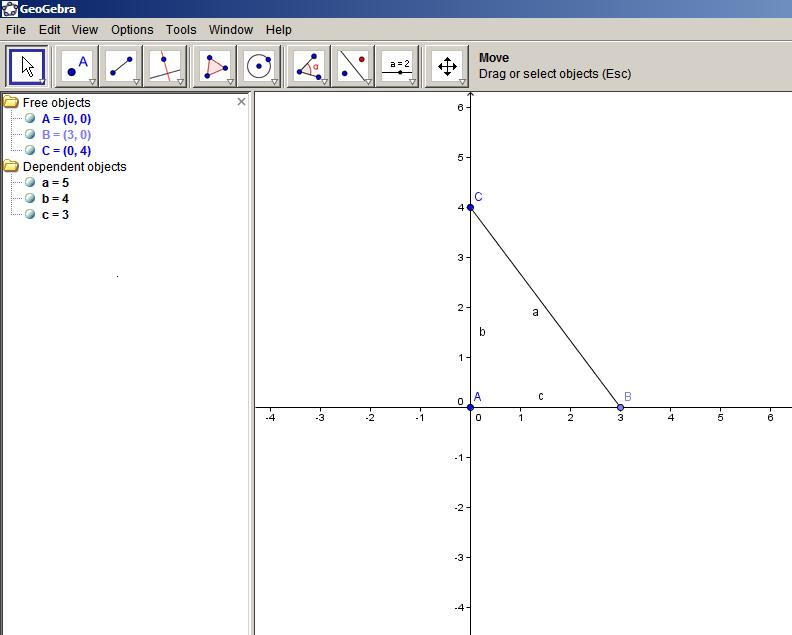Pythagoras theorem with the “standard” DGS (GeoGebra).

### Fig. 1

Pythagoras theorem with the “standard” DGS (GeoGebra).

Ancient Greek mathematicians introduced formalism in mathematics and even an axiomatic construction of geometry (Euclides’ Elements). It is well known that they knew and used Pythagoras theorem in a formal way.

Let us observe how the DGS Geometry Expressions can deal with Pythagoras theorem in an abstract way (Figure 2). After drawing point A, the constraint that $A = (0, 0)$ is added. After drawing point $B$ and point $C$, that their coordinates are $(0, c)$ and $(b, 0)$ (respectively  ) are added constraints (this way $B$ lies on the $y$ axis and $C$ lies on the $x$ axis). If we ask Geometry Expressions to measure the length of the diagonal of triangle $ABC$, $\sqrt{b^2+c^2}$ is obtained (the diagonal of the triangle depends on parameters $b$ and $c$, symbolic expressions that this DGS can directly manipulate).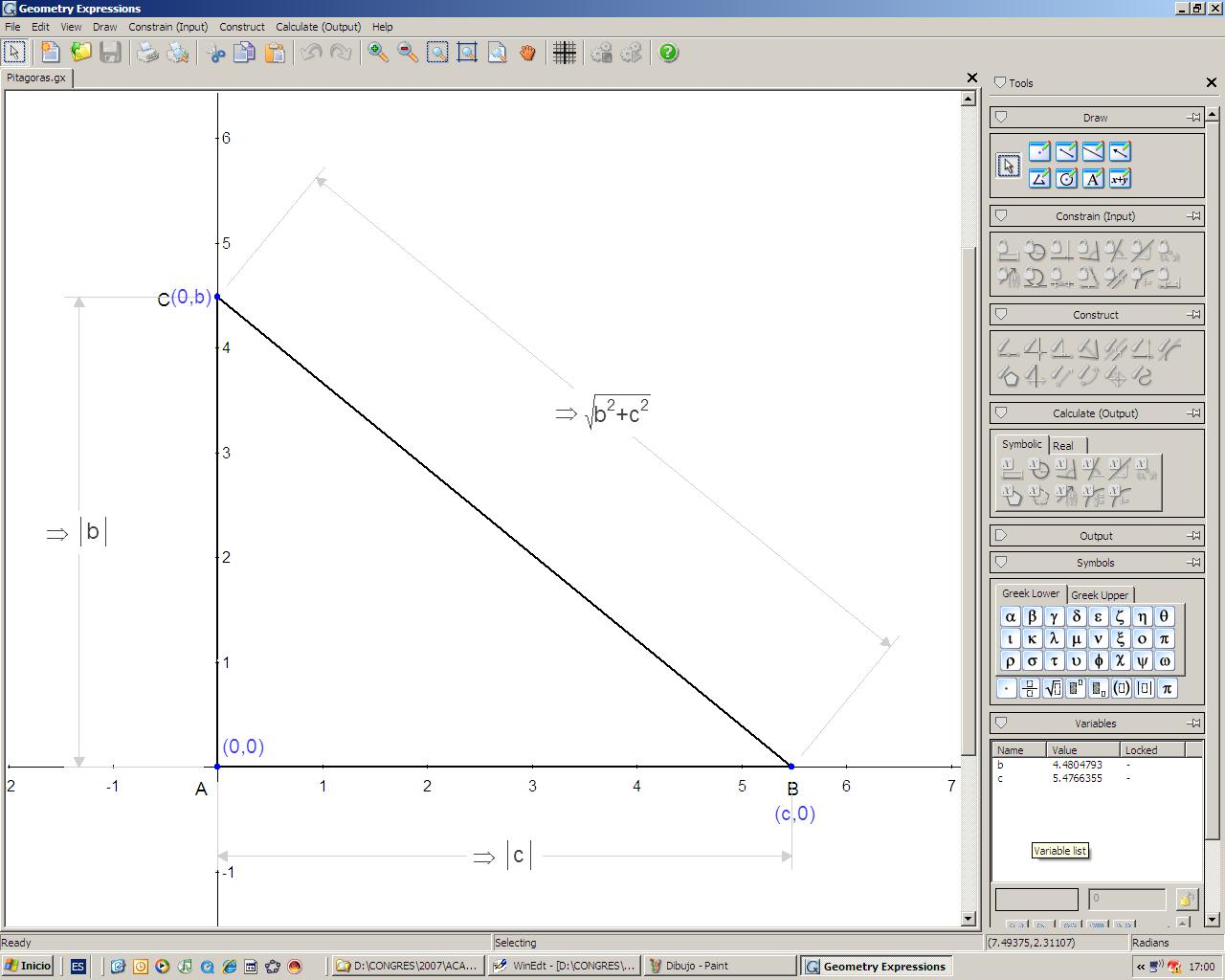Pythagoras theorem with Geometry Expressions.

### Fig. 2

Pythagoras theorem with Geometry Expressions.

Uniquely among DGSs, Geometry Expressions has been designed as a constraint-based system, a feature that enables a direct access to the symbolic representations of geometry problems, as demonstrated in the previous example, and therefore open the road for more general results.

#### 2.1.2 Proving the Existence of the Baricenter of a General Triangle just Typing One Single Line of Code Using The Geometer’s Sketchpad v.3 and paramGeo Package

In a standard DGS like The Geometer’s Sketchpad v.3  it is very easy to draw a triangle and its baricenter (Figure 3). The numerical coordinates of its baricenter can be obtained (at any time) just clicking with the mouse on this point. This simple notification is all we can obtain from all the well-known DGSs: obtaining a proof of the concept is not possible directly from such an output. However, this limitation can be overcome with the help of an external Maple package (paramGeo), by processing a description of the construction, as shown below.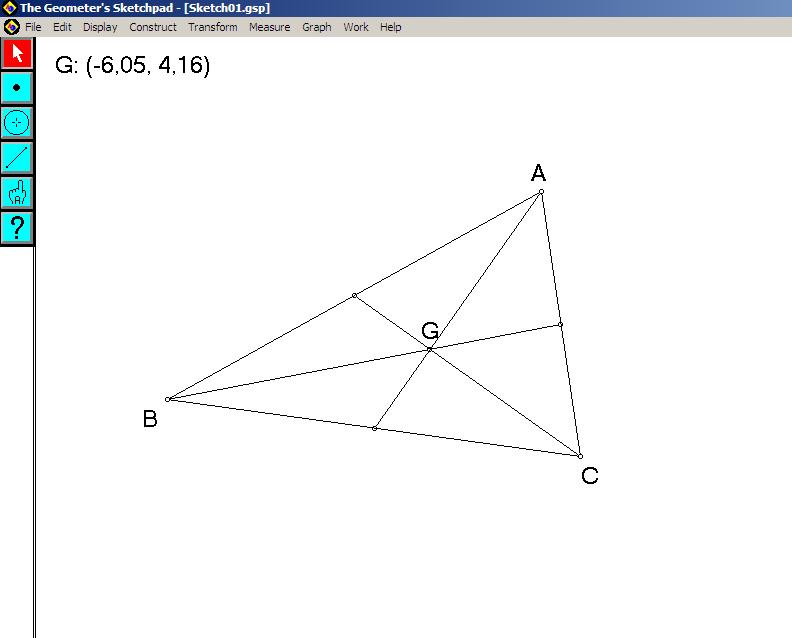Baricenter of a triangle with the “standard” DGS The Geometer’s Sketchpad.

### Fig. 3

Baricenter of a triangle with the “standard” DGS The Geometer’s Sketchpad.

The geometrical constructions produced with The Geometer’s Sketchpad are denoted “sketches”. In The Geometer’s Sketchpad (GSP) v.3 it is possible to obtain beautiful, almost natural language text “scripts”, that describe the geometric configuration and can be automatically created when building a “sketch” (see Figure 4).

These “scripts” can be automatically translated into Maple or Derive code by paramGeo’s translator. Note that these “scripts” were unfortunately abandoned in GSP v.4 , so when working with GSP v.4, paramGeo relies on the output produced by JavaSketchPad, a feature used to export geometric constructions on a web page.

Parameters are automatically associated by paramGeo’s translator to the coordinates of the points under “Given:” (Figure 4), unlike the derived points defined under “Steps:”, which coordinates are expressed using algebraic expressions in the coordinates of the given points.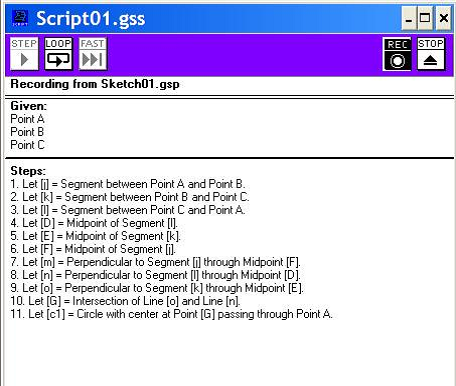A The Geometer’s Sketchpad v.3 “script” corresponding to the construction of the circumcenter of a triangle.

### Fig. 4

A The Geometer’s Sketchpad v.3 “script” corresponding to the construction of the circumcenter of a triangle.

For instance, the “script” corresponding to the construction of the baricenter of a triangle is:

        baric.gss
Given:
Point A
Point B
Point C
---------------
Steps:
1. Let [j] = Segment between Point A and Point B.
2. Let [k] = Segment between Point B and Point C.
3. Let [l] = Segment between Point C and Point A.
4. Let [D] = Midpoint of Segment [l].
5. Let [E] = Midpoint of Segment [k].
6. Let [F] = Midpoint of Segment [j].
7. Let [m] = Segment between Point A and Midpoint [E].
8. Let [n] = Segment between Midpoint [D] and Point B.
9. Let [I] = Intersection of Segment [n] and Segment [m].
10. Let [o] = Segment between Midpoint [F] and Point C.



that is automatically translated by paramGeo into a set of Maple’s instructions  :

            # Sketchpad to Maple automatic translation
# BARIC3.txt -> BARIC3.mpl
#BARIC3.gss
#
#Given:
A:=point(A_x, A_y);
B:=point(B_x, B_y);
C:=point(C_x, C_y);
#---------------
#Steps:
j:=segment(A, B);
k:=segment(B, C);
l:=segment(C, A);
D_:=midpoint(l);
E:=midpoint(k);
F:=midpoint(j);
m:=segment(A, E);
n:=segment(D_, B);
I_:=intersection(n, m);
o:=segment(F, C);


These geometric expressions can be used directly in Maple after loading a geometric package included with paramGeo. Then, to prove (not only to check) that the baricenter exists, only that the coordinates of $I$ (algebraic expressions in $Ax$, $Ay$, $Bx$, $By$, $Cx$, $Cy$) satisfy the equation of the line containing segment $o$ has to be checked with Maple (so it only requires the user to type one single line of code!).

### 2.2 Toward Standardization of the Connection of DGSs and CASs

The authors of GDI and webDiscovery  are working at connecting 2D DGSs and CASs using (and expanding) the standard OpenMath. In the future, any 2D DGS with the possibility to export the constructions in OpenMath format will be able to access any system that admits OpenMath as input, e.g. the package specialized in geometric discovery [18,29] webDiscovery (Figure 5).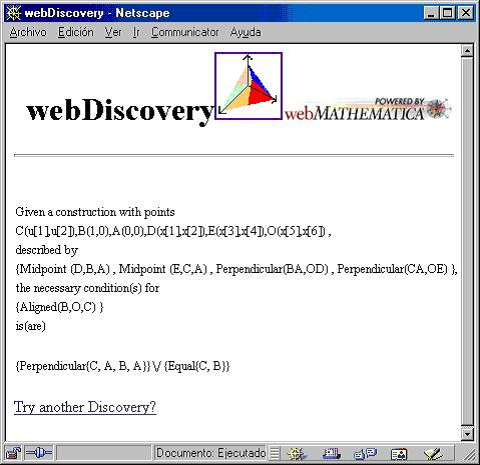Performing geometric discovery with webDiscovery.

### Fig. 5

Performing geometric discovery with webDiscovery.

## 3 3D DGSs – CASs Connection

### 3.1 3D DGSs – CASs Connection Preliminaries

Roughly speaking, there are two kinds of methods in mechanical theorem proving and discovery in geometry:

• methods based on the use of Gröbner bases [7,23],
• Wu’s method [9,46,47].

We have been working on these topics for several years (using both kinds of methods) [32,36].

We have implemented in the CAS Maple a package for 3D geometry, param-Geo3D, that we have successfully used to find (and prove) some new (!) 3D geometric theorems, such as:

• a version of Desargues theorem with tetrahedrons [38,39 ],
• a weak version of Pappus theorem [39,41] (see Figure 6),
• a 3D-extension of Steiner chains problem ,
• a 3D extension of Pascal theorem .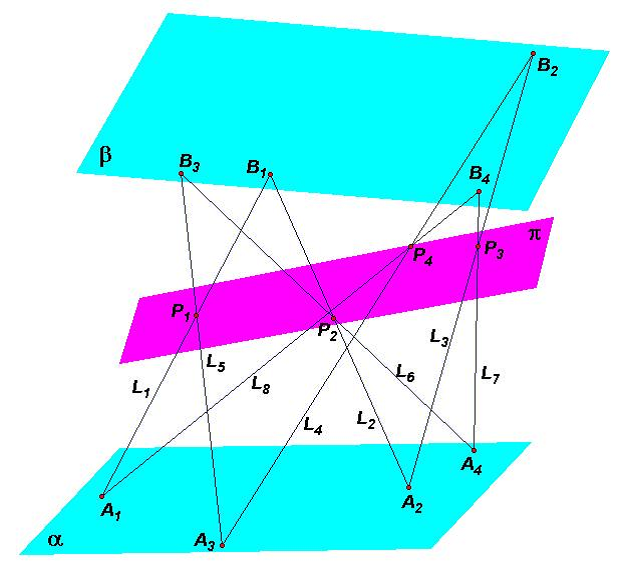Configuration of a weak version of Pappus theorem in 3D.

### Fig. 6

Configuration of a weak version of Pappus theorem in 3D.

It has to be noted that, in all these works, the details of the geometric configurations had to be manually introduced into Maple. This was due to the small number of suitable 3D-DGS systems available and to the fact that none of them would support any form of connection with CAS.

But a joint effort by the authors of this paper led to extending the features of Calques3D so that Mathematica-style and Maple-style versions of the history of a construction (Figure 8) could be exported.

Calques3D is a 3D DGS that shares most of the features of traditional DGSs; It supports the drawing of:

• points (see Figure 7), segments, lines, planes, spheres, . . .
• a point on a line, on a plane, on a sphere, . . .

and the construction of:

• the midpoint of a segment, the parallel line to a line through a point, the normal line to a line through a point, the perpendicular plane to a line through a point, . . .
• the intersection of two lines, of a line and a plane, of two spheres, . . .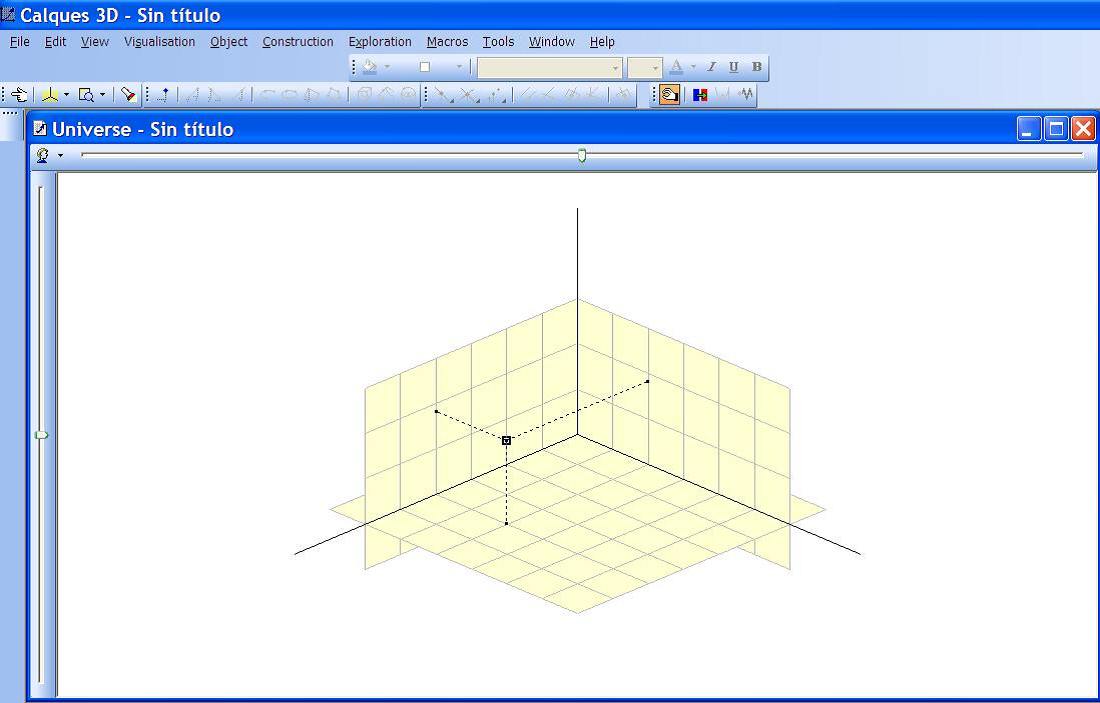Drawing a point in Calques3D.

### Fig. 7

Drawing a point in Calques3D.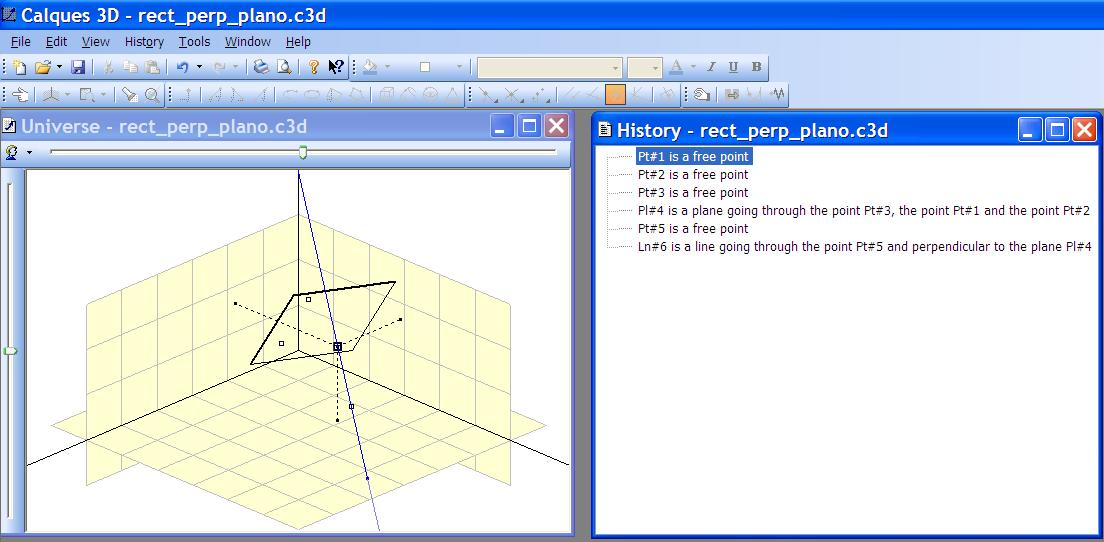Obtaining the “history” of a Calques 3D construction: perpendicular line to a plane, the latter defined by three non-collinear points.

### Fig. 8

Obtaining the “history” of a Calques 3D construction: perpendicular line to a plane, the latter defined by three non-collinear points.

Let us analyze what Calques3D history files really provide. For instance, if we draw three free points, the plane through them and a point lying on this plane, and we export the history file to Maple, we obtain something like:

            > Range(-0.5,4.9,-0.9,4.9,-0.5,0.5);
> FreePointD(Pt1,3,0,0);
> FreePointD(Pt2,2,3,0);
> FreePointD(Pt3,4,4,0);
> PlaneD(Pl4,Pt1,Pt2,Pt3);
> PointOnPlane(Pt5,Pl4);


Its first line is related to the visible part of the 3D space. The following three lines declare the first three objects to be points (as their names begin with a Pt) and include their numeric coordinates. The following line includes the description of the fourth object: the plane (as its name begins with a Pl) passing through points Pt1, Pt2 and Pt3. The last line describes the fifth object as a point lying on plane Pl4.

Therefore, a Calques3D history file is comparable to a The Geometer’s Sketchpad v.3 script: although it is in CAS syntax, it cannot include symbolic coordinates and equations with symbolic coefficients (i.e., parameters), so it is not useful for symbolic tasks.

This possibility of Calques3D to export constructions in CAS syntax was also used by Botana et al. in their system 3D-LD . It adopts our philosophy of software reuse [31,34] and also processes Calques3D output and use the resulting data as input to 3D-LD, that calls the CASs CoCoA and Mathematica for performing algebraic computations externally. Nevertheless this system was a prototype exclusively oriented to determining algebraic surfaces as geometric loci.

### 3.2 Connecting Calques3D with Maple

The key ideas behind this approach are:

• to use the preexisting capability of Calques3D to export the history of a construction in Maple-style syntax,
• to transform these history files, with a new “translator” designed ad hoc and written in Maple, so that symbolic coordinates and equations with symbolic coefficients can be inferred from the construction steps,
• to make the “translator” act as a mediator between the Maple-style orders exported by Calques 3D and those understood by Maple after loading paramGeo3D package,
• to adapt and reuse the existing Maple paramGeo3D package mentioned above

in order to develop a connection between Calques3D and Maple that can handle symbolic coordinates and equations with symbolic coefficients. This way many of Calques 3D’s “commands” (those related with the “3D ruler and compass geometry”) are now accessible for symbolic computation.

Our purpose in not only mechanical theorem proving and discovery in geometry, but a wider one:

• to explore 3D geometric problems that can be drawn in a 3D extension of “ruler and compass geometry”, and
• to automatically obtain their equations (for any required purpose, not only for mechanical theorem proving).

### 3.3 Implementation

In a nutshell, the connection process currently implemented is as follows: the geometric configurations are drawn with Calques3D, exported as a “history” file in Maple format, transformed by our specific package (so that symbolic coordinates and equations with symbolic coefficients can be processed) and finally used by Maple to perform any appropriate computations.

#### 3.3.1 The Translator File

The translator file translator.mpl contains procedures that convert the Calques3D history (in Maple format) into a specific paramGeo3D syntax.

Most of them are very simple. For instance, Calques3D’s command SegmentD calls paramGeo3D’s segment command and only has to consider that param- Geo3D works in projective coordinates and that n (the name of the object) is a global variable in paramGeo3D:

        > SegmentD:=proc(n,P,Q)
>   n:=subs(x0=1,segment(P,Q));
> end:


The reason for considering x0 = 1 is that P and Q cannot be points at infinity, as they have been input as free points using Calques3D.

The reason for paramGeo3D to work with projective coordinates is that it is often convenient in mechanical theorem proving in geometry to work with projective coordinates (for instance, degenerate cases related to an intersection point that vanishes when the two intersection lines become parallel, disappear ). However, a command in the paramGeo3D package allows to obtain the affine coordinates of a point, so that the use of projective coordinates can be kept hidden to the user.

For some constructions, like perpendicular lines, the translation is not so straightforward. In this case, Calques3D’s command PerpendicularLine includes only the name of the new line (n) and references to the base objects of the construction, a point (P1) and a line (L1). The translator calculates the pedal point of the perpendicular to L1 through P1 (using paramGeo3D commands) and assigns to variable n the line through P1 and the pedal point:

        > PerpendicularLine:=proc(n,P1,L1)
>   local Pperp,PenRec;
>   Pperp:=perpendicular(L1,P1);
>   PenRec:=intersection(L1,Pperp);
>   n:=subs(x0=1,line(P1,PenRec));
> end:


Finally, the family of intersection commands has been carefully analyzed. For instance, when intersecting planes, one algebraic object is returned by Calques3D history file:

        > IntersectionPlanes:=proc(n,PL1,PL2)
>   n:=subs(x0=1,intersection(PL1,PL2));
> end:


meanwhile when intersecting a line and a sphere, two algebraic objects (which would coincide when the line is tangent to the sphere) are returned by Calques3D history file, and they have to be distinguished:

        > Intersection1LineSphere:=proc(n,S1,L1)
>   local PtInt;
>   PtInt:=intersection(S1,L1);
>   n:=map(rhs,solve(PtInt,[x1,x2,x3]));
> end:

> Intersection2LineSphere:=proc(n,S1,L1)
>   local PtInt;
>   PtInt:=intersection(S1,L1);
>   n:=map(rhs,solve(PtInt,[x1,x2,x3]));
> end:


#### 3.3.2 The paramGeo3D Package

As said above, paramGeo3D package  had already been used by the authors in automatic theorem proving and discovery . In those works we had to directly type in Maple the details of the geometric configurations, instead of drawing the construction with the 3D DGS (as proposed in this article).

Let us include the code corresponding to plane procedure (that is called when translating Calques3D history command PlaneD), in order to get the flavor of paramgeo3D implementation:

        > plane:=proc(P::list,Q::list,R::list)
>   local d:
>   d:=plane_(P,Q,R):
>   if d=0 then
>       ERROR(‘the three points must be non-collinear‘)
>   fi:
>   constLess(d)=0
> end:

> plane_:=proc(P::list,Q::list,R::list)
>   det(matrix([[x0,x1,x2,x3],
>               [P,P,P,P],
>               [Q,Q,Q,Q],
>               [R,R,R,R]]))
>   end:


Procedure plane stores in variable d the equation of the plane through three points, P, Q, R (this polynomial is computed as a determinant by subprocedure plane_). If d is equal to zero, it returns an error message. Finally, using procedure constLess, the constants that are a common factor to all terms of polynomial d are suppressed.

#### 3.3.3 Calques 3D History Files Commands

The commands, currently available for translating the actions in Calques3D, are:

• FreePointD: draw a free point,
• SegmentD: draw a segment defined by its two endpoints,
• LineD: draw a line through two given distinct points,
• PlaneD: draw a plane through three given points,
• SphereD: draw a sphere (spherical surface), given its center and a point on the sphere,
• SphereRadiusD: draw a sphere (spherical surface), given its center and its radius (a segment),
• MidPoint: draw the midpoint of a given segment,
• ParallelLine: draw the parallel line to a given line or segment through a given point,
• NormalLine: draw the normal line to a given plane through a given point,
• NormalPlane: draw the normal plane to a given line through a given point,
• PerpendicularLine: draw the perpendicular line to a given line through a point not belonging to the given line,
• ParallelLine: draw the parallel line to a given line through a given point,
• IntersectionLinePlane: draw the intersection point of a given line and a given plane that are not parallel,
• IntersectionLines: draw the intersection point of two given lines that are coplanar but not parallel (i.e., that intersect exactly in a point),
• Intersection1LineSphere, Intersection2LineSphere: two points are obtained in the general case when an intersection line-sphere is computed by the 3D-DGS, and each of these two commands refer to one of them (in case of tangency, the two commands would refer to the same point),
• IntersectionPlanes: draw the intersection line of two given planes that are not parallel,
• IntersectionSphereSphere: draw the intersection of two given spheres (spherical surface); it can be a circle (circumference), a point or the empty set,
• PointOnSegment: draw a point lying on the given segment ,
• PointOnLine: draw a point lying on the given line,
• PointOnPlane: draw a point lying on the given plane,
• PointOnSphere: draw a point lying on the given sphere (spherical surface) (the names of these commands have been taken from Calques3D symbolic output for Maple).

Obviously, the commands above, as well as those commands in Maple’s param- Geo3D package, can be “manually” called from within a Maple session.

An overview of some possible applications of the 3D DGS-CAS connection developed is given in the next sections.

## 4 Applications of the Calques3D – Maple Connection provided by paramgeo3D

We first give two examples of a straightforward application of the process, obtaining the equations of geometrical objects that are known by Calques3D (Section 4.1) or even that cannot be handled by it (such as loci, Section 4.2).

Three examples of mechanical theorem proving will then be shown in Sections 4.3 to 4.5:

• the diagonals of a parallelepiped are concurrent,
• a 3D version of Desargues theorem (for triangles),
• a 3D version of Ceva and Menelaus theorems.

This latter example illustrates a case of Calques3D – Maple connection that cannot be performed by just using the standard output provided by Calques3D but needs some editing by the user.

Note that, in all the following examples, the Maple session should begin by loading:

• paramGeo3D Maple package, and
• the translator Maple package

### 4.1 Application I: Obtaining the Equations of a 3D Geometric Object known by Calques3D

A first straightforward application would be to obtain the generic equations of a geometric object directly constructed with Calques3D that is known by this 3D DGS, as shown in the next example.

Example 6 Obtaining the general equation of the tangent plane to a given sphere.

We want to calculate the general equation of the tangent plane to the sphere of center (0, 0, 0) and radius r through any point on it. We first draw the geometric configuration using Calques3D (Figure 9), by following these steps: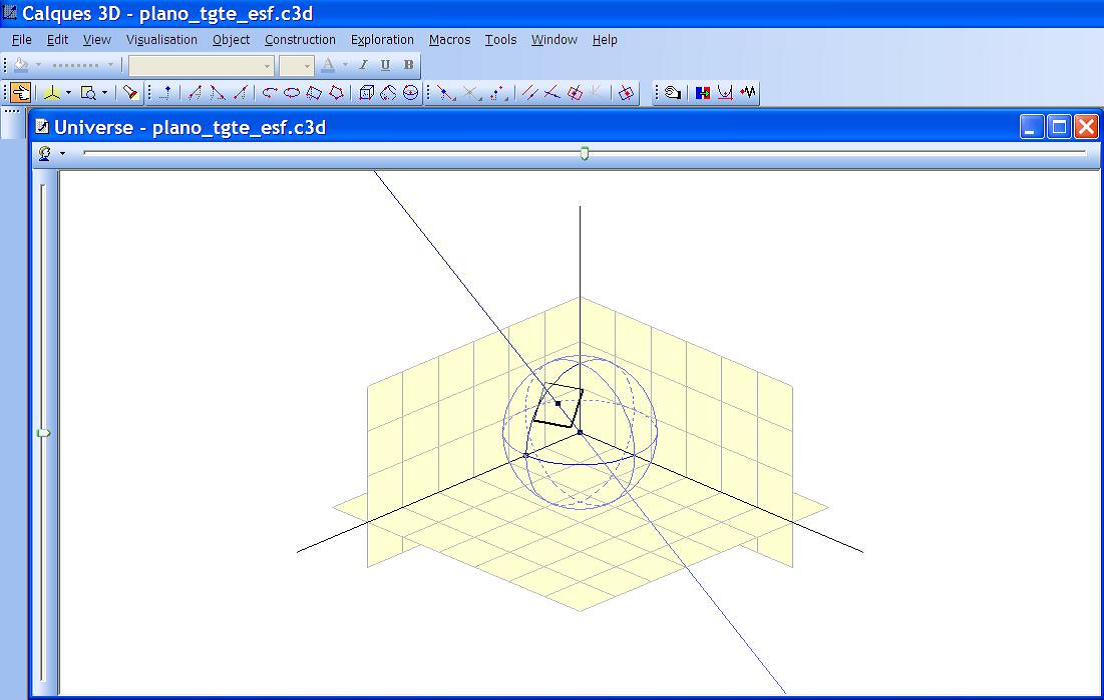The tangent plane to a sphere constructed with Calques3D.

### Fig. 9

The tangent plane to a sphere constructed with Calques3D.

• select magnetism  and draw point Pt1 = (0, 0, 0),
• deselect magnetism (so that the next point is really a free one, without restrictions),
• draw point Pt2 on the x axis,
• draw the sphere Sph3 of center Pt1 through Pt2,
• draw a point Pt4 on sphere Sph3,
• draw the line Ln5 through Pt1 and Pt4,
• draw the perpendicular plane (Pl6) to Ln5 through Pt4.

Then we can automatically obtain the corresponding “history” file:

        > Range(-2.0,2.0,-2.0,2.0,-2.0,2.0);
> FreePointD(Pt1,0,0,0);
> FreePointD(Pt2,23/15,0,0);
> SphereD(Sph3,Pt1,Pt2);
> PointOnSphere(Pt4,Sph3);
> LineD(Ln5,Pt1,Pt4);
> NormalPlane(Pl6,Pt4,Ln5);


Now we can start Maple and load the files. For the sake of simplicity, we rename the radius as r. Then we can directly ask Maple to return the equation of plane Pl6, the (general) tangent plane to the given sphere (# is used in Maple to add comments, that is, lines preceded by # are not executed):

        > # rename radius (i.e., Pt2x) as r
> Pt2x:=r:
> # get the equation of plane Pl6
> Pl6;

\begin{align}\begin{split} Pt4z \ Pt4x \ x1 + Pt4z \ Pt4y \ x2 + Pt4z^2 \ x3 \\ - \ Pt4x^2 \ Pt4z- Pt4z \ Pt4y^2 - Pt4z^3 = 0 \end{split}\end{align}

that is the general equation of the tangent plane to the given sphere through a generic point $Pt4 = (Pt4x, Pt4y, Pt4z)$ on it. Such a general expression would not be obtainable from a standard 3D DGS, like plain Calques3D.

It must be taken into account that point Pt4 was defined as lying on sphere Sph3. Consequently, the coordinates of $Pt4 = (Pt4x, Pt4y, Pt4z)$, must satisfy the equation of Sph3. The corresponding polynomial is automatically calculated and stored in list LREL by paramGeo3D package:

        > LREL;

$$[Pt4x2 + Pt4y2 + Pt4z2 − r2]$$

(as Sph3 is the sphere of center (0, 0, 0) and radius r, its equation is $x1^2+x2^2+x3^3-r^2=0$).

Let us check this general equation of the tangent plane in a simple example. The points on the sphere satisfying $x=0$ and $y=z$ are $(0,\frac{\sqrt{2}r}{2},\frac{\sqrt{2}r}{2})$ and $(0,-\frac{\sqrt{2}r}{2},-\frac{\sqrt{2}r}{2})$. They can be easily obtained with Maple:

        > # substitute Pt4x=0 and Pt4z=Pt4y in the first element of LREL
> subs(Pt4x=0,Pt4z=Pt4y,op(1,LREL)):
>
> # now solve the previous expression for Pt4y
> solve(%,Pt4y);

$$\frac{\sqrt{2}r}{2},-\frac{\sqrt{2}r}{2}$$

Let us consider, for instance, the first value, i.e, point $(0,\frac{\sqrt{2}r}{2},\frac{\sqrt{2}r}{2})$, and let us substitute these coordinates in the general equation of the tangent plane P16 obtained above, and solve it for x3:

        > subs(Pt4x=0,Pt4y=1/2*2^(1/2)*r,Pt4z=1/2*2^(1/2)*r,Pl6);

$$\frac{1}{2} r^2 x_2 + \frac{1}{2} x_3 r^2 - \frac{1}{2} \sqrt{2} r^3 = 0$$
        > x3=solve(%,x3);

$$x_3 = -x_2 + \sqrt{2} r$$

The result is trivially correct: the angle between this plane and a horizontal plane is 450 and it passes through $(0,\frac{\sqrt{2}r}{2},\frac{\sqrt{2}r}{2})$.

### 4.2 Application II: Obtaining the Equations of a Geometric Locus that is not a 3D Geometric Object known by Calques3D

It is also possible to obtain the equations of geometric loci constructed using Calques3D, as shown in the next example.

Example 7 Obtaining the equation of an ellipsoid.

We shall calculate now the equation of the ellipsoid of foci (0, 0, 0) and (1, 0, 0) and sum of distances to the foci equal to 2 (Figure 10). A modification for 3D of the common gardener’s method for drawing ellipses will be used.

The process followed to draw the ellipsoid is:

• choose magnetism and draw points Pt1 = (0, 0, 0) and Pt2 = (1, 0, 0) as foci and Pt3 = (0, 2, 0),
• draw segment Sg4 = Pt1Pt3,
• draw a point, Pt5, lying on this segment,
• draw the two segments that the previous point defines in segment Sg4,
• draw the two spheres defined by one of the foci and one of these two segments.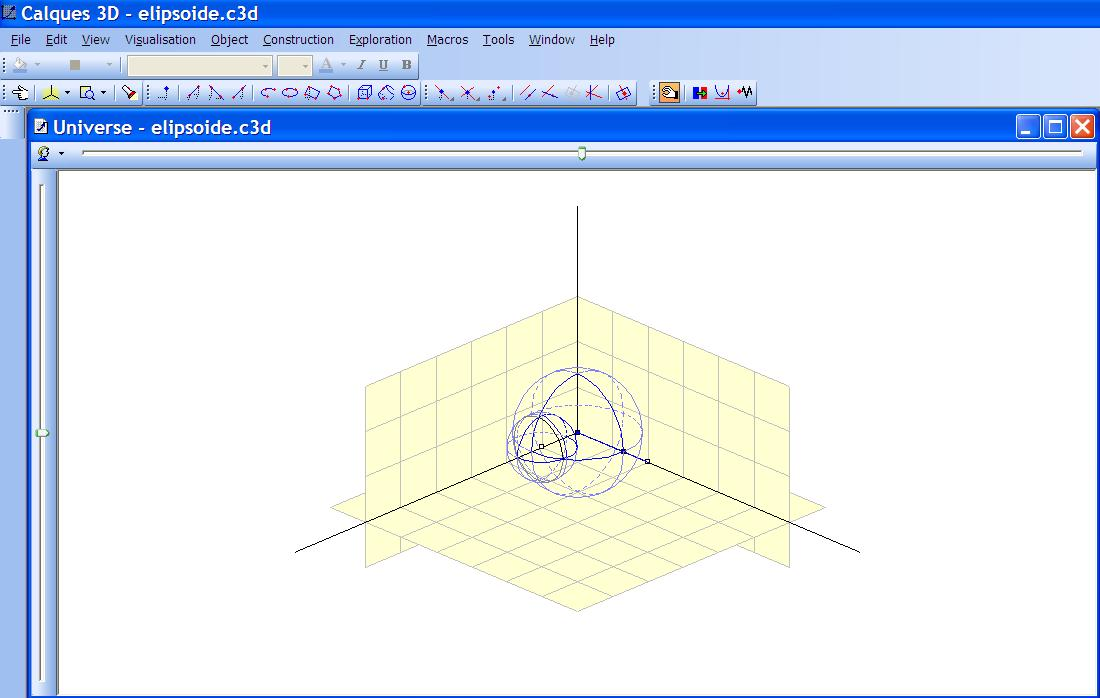Constructing an ellipsoid with Calques3D.

### Fig. 10

Constructing an ellipsoid with Calques3D.

The corresponding “history” file is:

        > Range(-1.9,2.1,-1.9,2.5,-1.9,1.9);
> FreePointD(Pt1,0,0,0);
> FreePointD(Pt2,1,0,0);
> FreePointD(Pt3,0,2,0);
> SegmentD(Sg4,Pt1,Pt3);
> PointOnSegment(Pt5,Sg4);
> SegmentD(Sg6,Pt1,Pt5);
> SegmentD(Sg7,Pt5,Pt3);
> IntersectionSphereSphere(Cr10,Sph8,Sph9);


As point Pt5 is defined as lying on segment Sg4 (itself contained in the y axis), it has one degree of freedom. Therefore, its coordinates could be written, for instance, as $Pt5:=(0,k1)$ ($0 \leq k1 \leq 2$). Let us observe that, when a Calques3D PointOnSegment command is detected by the translator, param- Geo3D’s pointOnSegment command is automatically called with $kn$ ($n \in \mathbb{N}$) as first input (n is initialized as 1 the first time pointOnSegment is used, and is increased by one each time pointOnSegment is called). Therefore, a k1 will appear as parameter in all computations involving the coordinates of Pt5.

So, paramGeo3D has automatically obtained as locus (without the user having to type anything):

        > Cr10;

$$[4 x2^2 + 4 x3^2 + 48 k1^2 + 9 - 48 k1, 2 x1 + 3 - 8 k1]$$

(that is, the intersection circle of the two spheres is expressed by paramGeo3D as the intersection of a cylinder and a plane, i.e., two algebraic varieties, both depending on a parameter, k1). Again, such an expression, that is key for obtaining the equation of the locus, would not be obtainable from a standard 3D DGS, like plain Calques3D.

We can now ask Maple to rewrite this parameter-depending description, by eliminating the parameter from the two equations, in order to obtain the equation of the locus (in the next line, Cr10 is converted from list to set using op, before applying eliminate):

> eliminate({op(Cr10)},k1);


$$[\{k1 = \frac{x_1}{4} + \frac{3}{8}\}, \{16 x_2^2 + 16 x_3^2 + 12 x_1^2 - 12 x_1 - 9\}]$$

Let us focus on the equation of the ellipsoid (the second element). It can be written:

        > # order the element of the second element of the previous
> # output w.r.t. the pure lexicographic order, being x1>x2>x3
> sort(op(op(2,%)),[x1,x2,x3],plex);


$$12 x_1^2 - 12 x_1 + 16 x_2^2 + 16 x_3^2 - 9$$

so the equation of the ellipsoid can be written:

> %/12=0;


$$x_1^2 - x_1 + \frac{4}{3} x_2^2 + \frac{4}{3} x_3^2 - \frac{3}{4} = 0$$

We can now plot the ellipsoid with Maple using command implicitplot3d (Figure 11).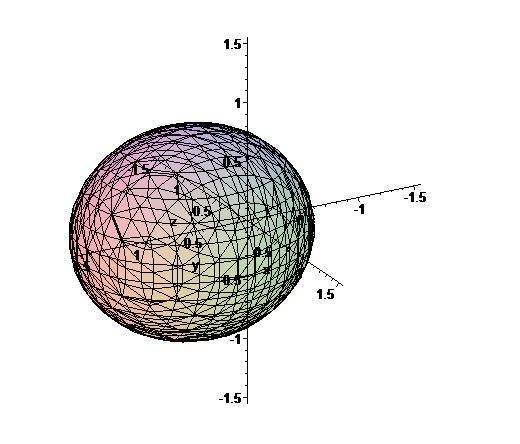Drawing with Maple an ellipsoid constructed with Calques3D.

### Fig. 11

Drawing with Maple an ellipsoid constructed with Calques3D.

### 4.3 Application III: Mechanical Theorem Proving in 3D Geometry

Let us begin with a very simple case.

Example 8 The diagonals of a parallelepiped are concurrent.

We first draw the geometric configuration (Figure 12) and can afterwards automatically obtain the corresponding “history” file (that is not included for the sake of brevity).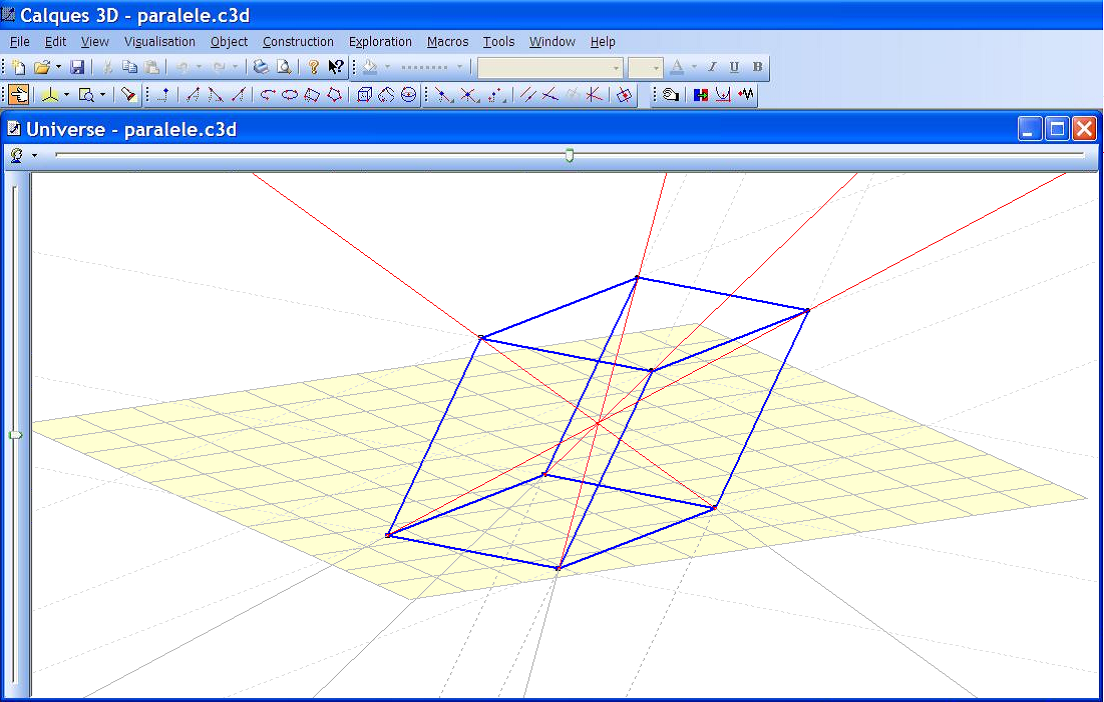Drawing a parallelepiped and its diagonals with Calques3D (some lines have be hidden to make the figure easier to visualize).

### Fig. 12

Drawing a parallelepiped and its diagonals with Calques3D (some lines have be hidden to make the figure easier to visualize).

The process followed to draw the parallelepiped is:

• draw three different points on plane z = 0 and a fourth point outside this plane (they are four vertices of the parallelepiped, and, if chosen this way, the pallelepiped, when completed, is completely general and cannot be a degenerated one),
• complete a parallelogram from the three points on plane z = 0 (drawing parallel lines and intersecting them),
• complete a parallelepiped from the parallelogram on plane z = 0 and the point outside this plane (drawing parallel lines and intersecting them),
• draw the four diagonals of the pallelepiped.

Now we can check with Maple that the intersection point of two of the four diagonals (Pt23), which coordinates have been automatically provided by the “history” file, belongs to the other two diagonals, (Ln24 and Ln25) –all these objects are defined in the “history” file.

We shall use paramGeo3D’s command isPlaced to check it. This command receives as input a point and an object. The latter is:

1. either directly defined by its equation, as is the case for a plane (from the algebraic point of view, the object is a surface),
2. or given as the intersection of two objects directly defined by their equations, as is the case for a line –given as the intersection of two planes (from the algebraic point of view, the object is a curve, defined as the intersection of two surfaces)

and returns as output the result of substituting the coordinates of the point in the equation(s) of the object. Therefore, the given point lies on the given object if and only if (respectively):

1. 0 is obtained
2. [0, 0] is obtained

Let us check our present example:

        > isPlaced(Pt23,Ln24);
[0, 0]
> isPlaced(Pt23,Ln25);
[0, 0]


As [0, 0] is obtained in both cases, the point lies on the other two diagonals too.

We would like to stress that, after loading paramGeo3D and the translator Maple packages and the “history” file in Maple format, all what the user had to type in the Maple session to obtain the formal proof were the two lines above!

### 4.4 Application IV: Checking that Certain Coordinates or Equations do Correspond to a Locus

Determining geometric loci can be linked to mechanical theorem proving in geometry. For instance, we have proven in Example 8 that the diagonals of a parallelepiped are concurrent. Now we can use the Calques3D – Maple connection provided by paramgeo3D to check that the coordinates of such intersection point are the average of the coordinates of the vertices of the parallelepiped.

Example 9 The coordinates of the common point to the diagonals of a parallelepiped are the average of the coordinates of its vertices.

With the notation of Example 8 (this is a continuation of it), we have to check that the coordinates of Pt23 are the average of the coordinates of Pt1, Pt2, Pt3, Pt4, Pt10, Pt15, Pt18, Pt19.

The coordinates of Pt23 are  :

    > Pt23;

$$Pt23 := [1, -\frac{Pt1x}{2} + \frac{Pt2x}{2} + \frac{Pt4x}{2} + \frac{Pt3x}{2} ,\\ \frac{Pt1y}{2} + \frac{Pt4y}{2} + \frac{Pt2y}{2} - \frac{Pt3y}{2} ,\\ \frac{Pt4z}{2} ]$$

Observe that not all Pt1, Pt2, Pt3, Pt4, Pt10, Pt15, Pt18, Pt19 appear in the expression of Pt23 above. That happens because there are dependencies between the coordinates of these points (i.e., they are not all free points –due to the parallelism declarations). This is why the result is not trivial, and some computations have to be made.

So let us compare the (affine) coordinates of Pt23 with the average of the (affine) coordinates of the vertices  :

    > # x coordinate
> op(2,Pt23);

$$-\frac{Pt1x}{2} + \frac{Pt2x}{2} + \frac{Pt4x}{2} + \frac{Pt3x}{2}$$
    > (Pt1+Pt2+Pt3+Pt4+Pt10+Pt15+Pt18+Pt19)
/8;

$$-\frac{Pt1x}{2} + \frac{Pt2x}{2} + \frac{Pt4x}{2} + \frac{Pt3x}{2}$$

So the result holds for the first (affine) coordinate. The same happens for the other two coordinates, and therefore the result is true.

### 4.5 Application III: Mechanical Theorem Proving in 3D Geometry (Continued)

In Example 8 a very simple example of mechanical theorem proving in geometry was presented. As will be shown in Examples 10 and 11, the symbolic connection between Calques3D and Maple provided by paramGeo3D allows to prove, with just some mouse clicks and typing one or two lines of Maple code, non-trivial 3D geometric theorems.

Example 10 A 3D version of Desargues theorem (for triangles).

The steps followed in the construction of the configuration are the following (see Figure 13):

• draw a non-degenerated triangle, which three vertices are outside plane z = 0, first (directly with side-lines),
• choose a point that is neither on the plane containing the triangle nor on plane z = 0,
• project the vertices of the triangle on plane z = 0 (as the vertices of the triangle of the first step are outside this particular plane, there is no possibility of encountering a degenerate case; moreover, there is no loss of generality by projecting on that plane –this plane is only chosen in order to simplify the equations obtained),
• construct the side-lines through the projections of the vertices,
• intersect each pair of corresponding side-lines (3 points are obtained),
• construct the line through 2 of these points,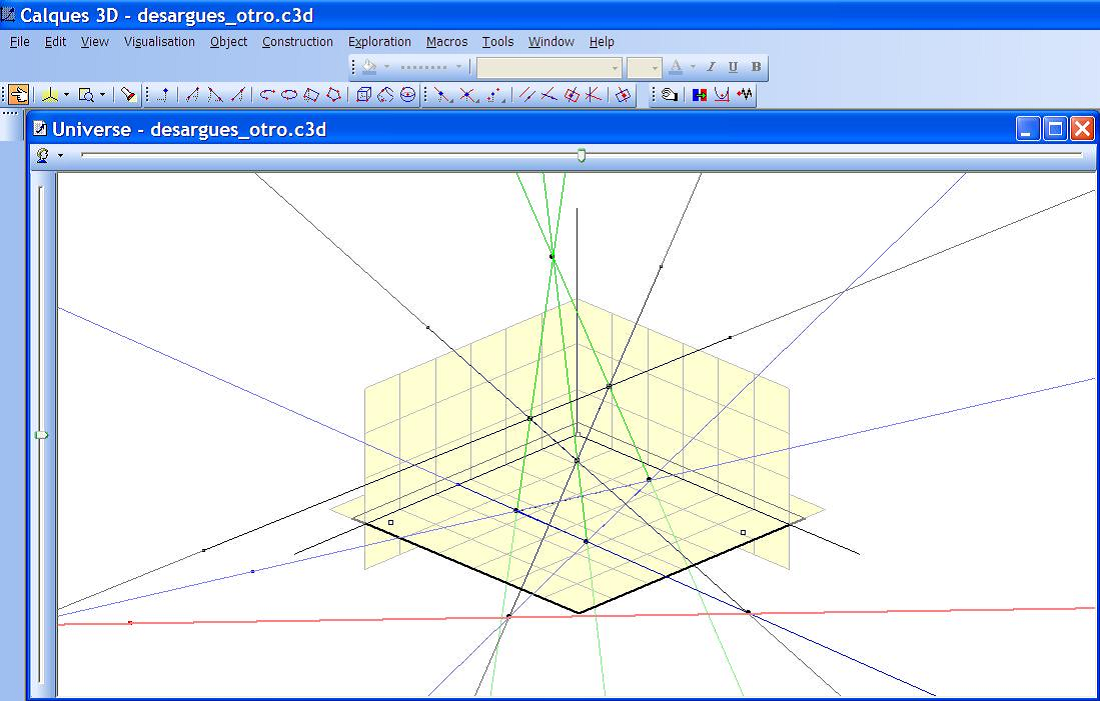A 3D version of Desargues theorem with triangles.

### Fig. 13

A 3D version of Desargues theorem with triangles.

and the thesis is that this line passes through the third point.

The history file provided by Calques3D is not included for the sake of brevity.

The three points obtained in the penultimate step of the construction are Pt23, Pt26 and Pt29 and the line through Pt26 and point Pt23 is denoted Ln30. Now we only have to check with Maple that point Pt29 lies on line Ln30:

    > isPlaced(Pt29,Ln30);


As [0, 0] has been returned, Pt23 lies on Ln30 (because it lies on the two surfaces defining line Ln30), so the result is true in general.

We shall finally include an example of mechanical theorem proving of a different kind, where a rate between segments is involved. This makes it necessary to exchange by hand PointOnLine by PointOnLineRate in some lines of the Calques3D history file, in order to make use of paramGeo3D’s command pointOnLineRate.

Example 11 A 3D version of Ceva and Menelaus theorems.

The steps followed in the construction of the configuration (see Figure 14) are the following:

• draw Pt1 = (0, 0, 0), Pt2 = (1, 0, 0) and Pt3 on plane z = 0, and Pt4 outside plane z = 0,
• draw consecutive side-lines of the tetrahedron, for instance, $\overline{Pt1Pt2}$, $\overline{Pt2Pt3}$, $\overline{Pt3Pt4}$ and $\overline{Pt4Pt1}$ (important: select the points in the order specified, e.g., first Pt4 and then Pt1),
• draw a point on each of these side-lines: Pt9, Pt10, Pt11, Pt12 (respectively),
• draw the plane Pl13 through the points Pt9, Pt10, Pt11.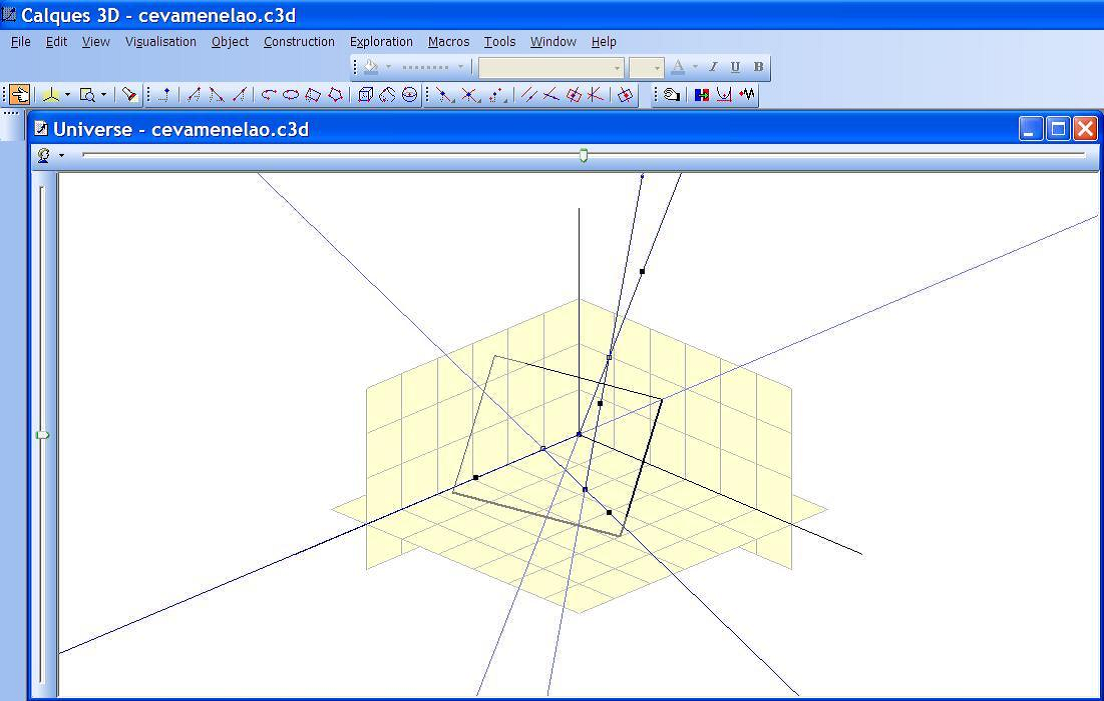A 3D version of Ceva and Menelaus theorems.

### Fig. 14

A 3D version of Ceva and Menelaus theorems.

Then, if the ratios (m, n, p, q) of the two segments in which the points Pt9, Pt10, Pt11 and Pt12 divide the corresponding segment verify m· n · p · q = 1, the four points are coplanar.

The history file provided by Calques3D is:

    > Range(-0.5,3.7,-1.2,4.4,-0.8,6.1);
> FreePointD(Pt1,0,0,0);
> FreePointD(Pt2,1,0,0);
> FreePointD(Pt3,79/45,129/67,0);
> FreePointD(Pt4,69/68,177/95,143/54);
> LineD(Ln5,Pt1,Pt2);
> LineD(Ln6,Pt2,Pt3);
> LineD(Ln7,Pt3,Pt4);
> LineD(Ln8,Pt4,Pt1);
> PointOnLineRate(m,Pt9,Sg5); #PointOnLine(Pt9,Ln5); #by hand
> PointOnLineRate(n,Pt10,Sg6); #PointOnLine(Pt10,Ln6); #by hand
> PointOnLineRate(p,Pt11,Sg7); #PointOnLine(Pt11,Ln7); #by hand
> PointOnLineRate(q,Pt12,Sg8); #PointOnLine(Pt12,Ln8); #by hand
> PlaneD(Pl13,Pt9,Pt10,Pt11);


where we have manually substituted the PointOnLine command by Point- OnLineRate command, in order to specify the ratios (m, n, p, q) of the two segments in which the point divides the corresponding segment.

Plane Pl13 is defined as the plane passing through points Pt9, Pt10, Pt11. Let us check with Maple that the theorem holds, i.e., that if

$$m \cdot n \cdot p \cdot q=1 \Leftrightarrow m = \frac{1}{n \cdot p \cdot q}$$

then Pt12 lies on plane Pl13:

        > isPlaced(Pt12,Pl13);

$$Pt3y \ Pt4z - m \ n \ q \ Pt4z \ p \ Pt3y$$
        > subs(m=1/(n*p*q),%);

$$0$$

Therefore the theorem has been proven just adding four lines of code to the history file and typing two lines of code.

## 5 Conclusions

This work has a didactic application in geometric problems exploration. Nevertheless, its main interest is to provide a convenient way to explore and automatically obtain the corresponding algebraic or numerical data when dealing with a 3D extension of “ruler and compass geometry”, which has a wider scope than only educational purposes (e.g., mechanical theorem proving in geometry, geometric discovery, geometric loci finding...). As far as we know, apart from the restricted prototype 3D-LD, there is no comparable software for 3D!

We would like to underline that, as shown in Example 11, manually editing the output of the DGS is sometimes needed. Further developments have to be carried out in the DGS side to completely characterize these cases. But both options are not mutually exclusive: both may need to be done in some cases. To summarize, the export, translation and treatment of the construction history, in order to obtain a format usable by Maple, and involving symbolic coordinates and equations with symbolic coefficients, has now been completed and expanded to cover all the “ruler and compass geometry”.

As future work, Calques 3D’s author is now working on improving the user interface with ParamGeo3D, using the openMaple API [70 ,25], which allows direct calls to the Maple kernel from external programs. This will remove the need, as described in this paper, of working alternately with the two systems and saving/loading an intermediary file. But, in the long-term, it will also open the possibility for supporting the other part of the DGS-CAS communication: being able to immediately use the output of the CAS for modifying or constructing new elements in a configuration (as Geometry Expressions is already able to do in the 2D case).

## 6 Acknowledgments

This work was partially supported by the research projects MTM2004-03175 (Ministerio de Educación y Ciencia, Spain), UCM2005-910563 and UCM2006- 910563 (Comunidad de Madrid - Universidad Complutense de Madrid, research group ACEIA) and UCM2008-910563 (UCM - BSCH Gr. 58/08, research group ACEIA).

We would also like to thank the anonymous referees for their most valuable comments, that have really improved the article.

## References

1. L. Bazzotti, G. Dalzotto , L. Robbiano, Remarks on Geometric Theorem Proving, in: J. Richter-Gebert, D. Wang (editors), Proceedings of ADG 2000, Springer-Verlag, LNAI 2061, Berlin-Heidelberg, 2001, pp. 104–128.
2. F. Botana, 3D-LD: automatic discovery of 3D loci via the web (Technical Report), Department of Applied Mathematics, University of Vigo, 2002.
3. F. Botana, J.L. Valcarce, A dynamic-symbolic interface for geometric theorem discovery, Computers and Education 38/1-3 (2002) 21-35.
4. F. Botana, J.L. Valcarce, A software tool for the investigation of plane loci, Mathematics and Computers in Simulation 61/2 (2003) 141-154.
5. F. Botana, J.L. Valcarce, Automatic determination of envelopes and other derived curves within a graphic environment, Mathematics and Computers in Simulation 67/1-2 (2004) 3-13.
6. F. Botana, A web-based resource for automatic discovery in plane geometry, International Journal of Computers for Mathematical Learning 8/1 (2003) 109- 121.
7. B. Buchberger, Applications of Gröbner Bases in non-linear Computational Geometry, in: J.R. Rice (editor), Mathematical Aspects of Scientific Software, Springer-Verlag, 1988, pp. 59-87.
8. M. Bulmer, D. Fearnley-Sander, T. Stokes, The Kinds of Truth of Geometry Theorems, in: J. Richter-Gebert, D. Wang (editors), Proceedings of ADG 2000 Springer-Verlag, LNAI 2061, Berlin-Heidelberg, 2001, pp. 129–142.
9. C. Chou, Mechanical Geometry Theorem Proving, Reidel, 1988.
10. S.C. Chou, X.S. Gao, Z. Ye, Java Geometry Expert, in: Proceedings of the 10th Asian Technology Conference in Mathematics, 2005, pp. 78-84.
11. P. Conti, C. Traverso, Algebraic and Semialgebriac Proofs: Methods and Paradoxes, in: J. Richter-Gebert, D. Wang (editors), Proceedings of ADG 2000. Springer-Verlag, LNAI 2061, Berlin-Heidelberg, 2001, pp. 83–103.
12. R.M. Corless, Essential Maple, Springer-Verlag, New York Berlin Heidelberg, 1995.
13. A. Dolzmann, T. Sturm, V.Weispfenning, A New Approach for Automatic Theorem Proving in Real Geometry, J. of Automated Reasoning 21 (1998) 357–380.
14. K. Fuchs, M. Hohenwarter, Combination of Dynamic Geometry, Algebra and Calculus in the Software System GeoGebra, in: Computer Algebra Systems and Dynamic Geometry Systems in Mathematics Teaching Conference 2004, Pecs, Hungary, 2005.
15. X.S. Gao, J.Z. Zhang, S.C. Chou, Geometry Expert, Nine Chapters Publishers, 1998.
16. A. Heck, Introduction to Maple, Springer-Verlag, New York Berlin Heidelberg, 1996.
17. R.D. Jenks, R.S. Sutor, Axiom. The Scientific Computation System, Springer- Verlag, New York Berlin Heidelberg, 1992.
18. D. Kapur, J.L. Mundy, Wu’s method and its application to perspective viewing, in: D. Kapur, J.L. Mundy (editors), Geometric Reasoning, MIT Press, Cambridge, MA, 1989, pp. 15-36.
19. U. Kortenkamp, Foundations of Dynamic Geometry (PhD. Thesis), Swiss Fed. Inst. Tech. Zurich, 1999.
20. B. Kutzler, Introduction to DERIVE for Windows, BK-Teachware, Hagenberg, Austria, 1996.
21. B. Kutzler, Careful algebraic translations of geometry theorems, in: G.H. Gonnet (editor), Proceedings of ISSAC 89, ACM Press, Portland, Oregon, 1989, pp. 254-263.
22. B. Kutzler, V. Kokol-Voljc, DERIVE 5. The Mathematical Assistant for your PC, BK-Teachware, Hagenberg, Austria, 2000.
23. B. Kutzler, S. Stifter, On the application of Buchberger’s algorithm to automated geometry theorem proving, Journal of Symbolic Computation 2/4 (1986) 389–397.
24. M. MacCallum, F.Wright, Algebraic Computing with Reduce, Clarendon Press, Oxford, 1991.
25. Maplesoft: Maple User Manual. Maplesoft, 2005.
26. G. Rayna, REDUCE. Software for Algebraic Computation, Springer-Verlag, New York Berlin Heidelberg, 1987.
27. T. Recio, Cálculo Simbólico y Geométrico, Ed . Síntesis, 1998.
28. T. Recio, F. Botana, Where the Truth Lies (in Automatic Theorem Proving in Elementary Geometry), in: A. Laganá et al. (editors), Proceedings of ICCSA 2004 Springer-Verlag, LNCS 3044, Berlin-Heidelberg, 2004, pp. 761–770.
29. T. Recio, M.P. Vélez, Automatic Discovery of Theorems in Elementary Geometry, Journal of Automated Reasoning 23 (1999) 63-82.
30. A. Rich et al., DERIVE User Manual, Soft Warehouse, Honolulu, 1994.
31. E. Roanes-Lozano, Boosting the Geometrical Possibilities of Dynamic Geometry Systems and Computer Algebra Systems Through Cooperation, in: M. Borovcnik, H. Kautschitsch (editors), Technology in Mathematics Teaching. Proceedings of ICTMT-5, ¨obv & hpt, Schrifrenreihe Didaktik der Mathematik 25, Viena, 2002, pp. 335-348.
32. E. Roanes-Lozano, E. Roanes-Macías, Automatic Theorem Proving in Elementary Geometry with Derive 3, The International Derive Journal 3/2 (1996) 67-82.
33. E. Roanes-Lozano, E. Roanes-Macías, How Dynamic Geometry could Complement Computer Algebra Systems (Linking Investigations in Geometry to Automated Theorem Proving), in: Proceedings of the Fourth International Derive/TI-89/TI-92 Conference (CD-ROM), BK-Teachware, Hagenberg, Austria, 2000.
34. E. Roanes-Lozano, E. Roanes-Macías, M. Villar Mena, A Bridge Between Dynamic Geometry and Computer Algebra. Mathematical and Computer Modelling 37/9-10 (2003) 1005-1028.
35. E. Roanes-Lozano, E. Roanes-Macías, A Simple Geometric Theorem with a Constructive Configuration Whose Truthfulness Depends on the Base Field Considered, International J. of Computer Information Systems and IndustrialManagement Applications (IJCISIM) Spec.Vol. 1 (2008) 22–29 (URL: www.softcomputing.net/~ijcisim/)
36. E. Roanes-Macías, E. Roanes-Lozano, Nuevas Tecnologías en Geometría, Editorial Complutense, Madrid, 1994.
37. E. Roanes-Macías, E. Roanes-Lozano, Cálculos Matemáticos con Maple V.5, Ed. Rubi˜nos, Madrid (1999) (In Spanish).
38. E. Roanes-Macías, E. Roanes-Lozano, A method for outlining 3D problems in order to study them mechanically. Application to prove the 3D-version of Desargues, in: L. González-Vega, T. Recio (editors): Actas de los Encuentros de Algebra Computacional y Aplicaciones (EACA’2004). Universidad de Cantabria, 2004, pp. 237–242.
39. E. Roanes-Macías, E. Roanes-Lozano, A Maple Package for Automatic Theorem Proving and Discovery in 3D-Geometry, in: F. Botana, T. Recio (editors): Automated Deduction in Geometry, 6th International Workshop, ADG 2006. Lozano, 3D-extension of Steiner chains problem, Mathematical and Computer Modelling 45 (2007) 137–148.
40. E. Roanes-Macías, E. Roanes-Lozano, J. Fernández-Biarge, Extensión natural a 3D del teorema de Pappus y su configuración completa, Bol. Soc. “Puig Adam” 80 (2008) 38–56.
41. E. Roanes-Macías, E. Roanes-Lozano, J. Fernández-Biarge, Obtaining a 3D extension of Pascal theorem for non-degenerated quadrics and its complete configuration with the aid of a computer algebra system. RACSAM (Revista de la Real Academia de Ciencias Exactas, Físicas y Naturales, Serie A, Matemáticas) 103/1 (2009) 93-109.
42. P. Todd, Geometry Expressions: A Constraint Based Interactive Symbolic Geometry System, in: F. Botana, T. Recio (editors): Automated Deduction in Geometry, 6th International Workshop, ADG 2006. Springer-Verlag Lecture Notes in Artificial Intelligence 4689, Berlin Heidelberg New York, 2007, pp. 189–202
43. D. Wang, GEOTHER 1.1: Handling and Proving Geometric Theorems Automatically, in: F. Winkler (editor), Automated Deduction in Geometry, Lecture Notes in Artificial Intelligence 2930, Springer-Verlag, Berlin Heidelberg New York, 2004, pp. 194-215.
44. S. Wolfram, Mathematica. A System for Doing Mathematics by Computer, Addison-Wesley, Redwood City, CA (1991).
45. W. Wen-Tsun, On the decision problem and the mechanization of theorem proving in elementary Geometry, A.M.S. Contemporary Mathematics 29 (1984) 213-234.
46. W. Wen-Tsun, Some recent advances in Mechanical Theorem-Proving of Geometries, A.M.S. Contemporary Mathematics 29 (1984) 235–242.
47. –, The Geometer’s Sketchpad User Guide and Reference Manual v.3, Key Curriculum Press, Berkeley, CA, 1995.
48. –, The Geometer’s Sketchpad Reference Manual v.4, Key Curriculum Press, Emeryville, CA, 2001.
49. URL: http://www.wolfram.com
50. URL: http://www.maplesoft.com
51. URL: http://education.ti.com/educationportal/sites/US/productDetail/us derive6.html
52. URL: http://www.reduce-algebra.com/
53. URL: http://www.axiom-developer.org/
55. URL: http://maxima.sourceforge.net/
56. URL: http://cocoa.dima.unige.it
57. URL: http://www.singular.uni-kl.de/
58. URL: http://education.ti.com/educationportal/sites/US/productDetail/us cabri geometry.html
59. URL: http://www.keypress.com/x5521.xml
60. URL: http://cinderella.de/tiki-index.php
61. URL: http://www.geogebra.org/cms/
62. URL: http://www.calques3d.org
63. URL: http://pygeo.sourceforge.net
64. URL: http://www.mmrc.iss.ac.cn/gex/
65. URL: http://www.cs.wichita.edu/~ye/
66. URL: http://www.geometryexpressions.com/
67. URL: http://www-calfor.lip6.fr/~wang/epsilon
68. URL: http://www-calfor.lip6.fr/~wang/GEOTHER/
5. The treatment of degenerated cases is an important problem in automated theorem proving . Another important fact is that we sometimes draw and think in $\mathbb{R}^n$ and carry out the computations in $\mathbb{C}^n$ . Moreover, there are discussions and different opinions about what is a “true” theorem [1,8 ,11,13,28].